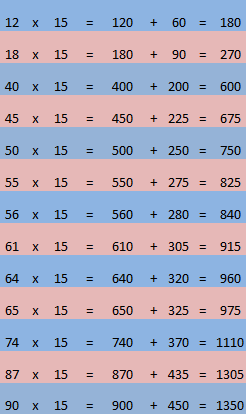Multiply by 15/ Finding 15% (0.15)

This is an other popular trick. A lot of the times called the Restaurant Tip because it is 15% of the total bill.

Obviously the number you will get when multiplying by 15  is 100 times bigger than what the 15% is.

To this you need to use a 3 step approach.
Let us use 34 for example

1)    Find what the number multiplied by 10 is.
This is very easy as you simply add a 0 at the end.
34×10 = 340

2)     Find what the number times 5 is.
To this fast find what that number times 10 is and divide by 2. We did this in the previous step.
340/2= 170

3)    Add these 2 numbers together
340+170=540

For finding 15% (0.15) of a number simply divide this number by 10.

View bellow a couple of examples